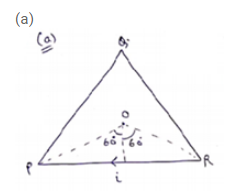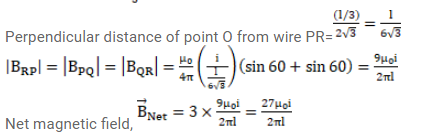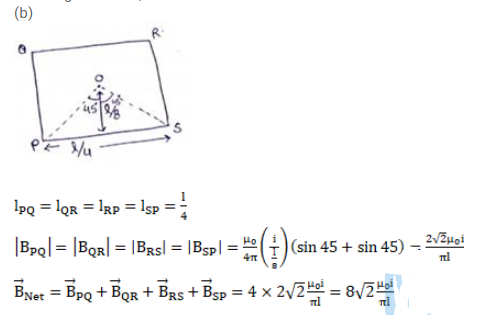# A wire of length I is bent in the form of an equilateral triangle

Question:

A wire of length I is bent in the form of an equilateral triangle and carries an electric current i.

(a) Find the magnetic field B at the center.

(b) If the wire is bent in the form of a square, what would be the value of B at the center?

Solution:$\mathrm{l}_{\mathrm{pQ}}=\mathrm{l}_{\mathrm{QR}}=\mathrm{l}_{\mathrm{RP}}=\frac{1}{3}$